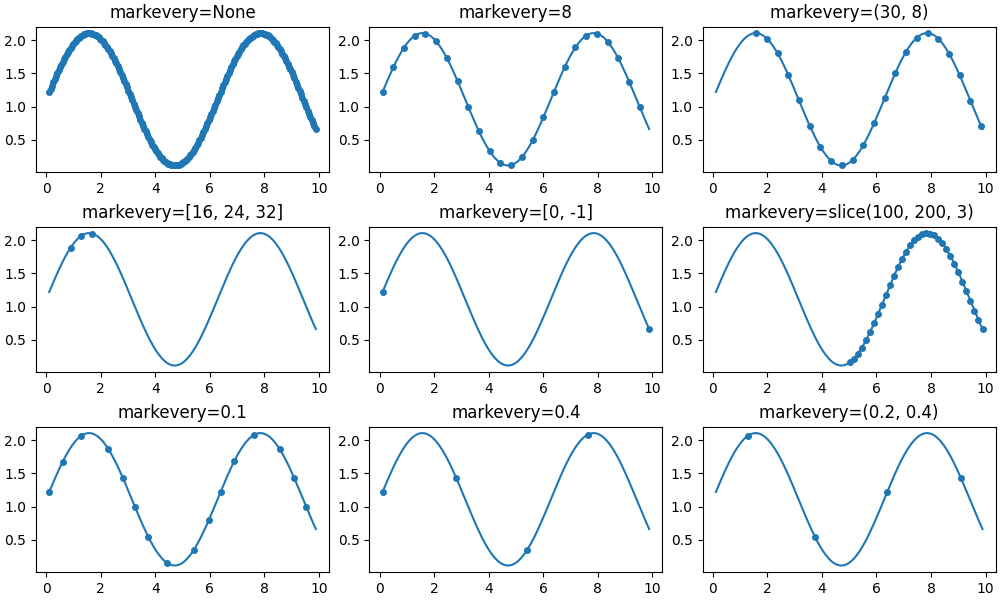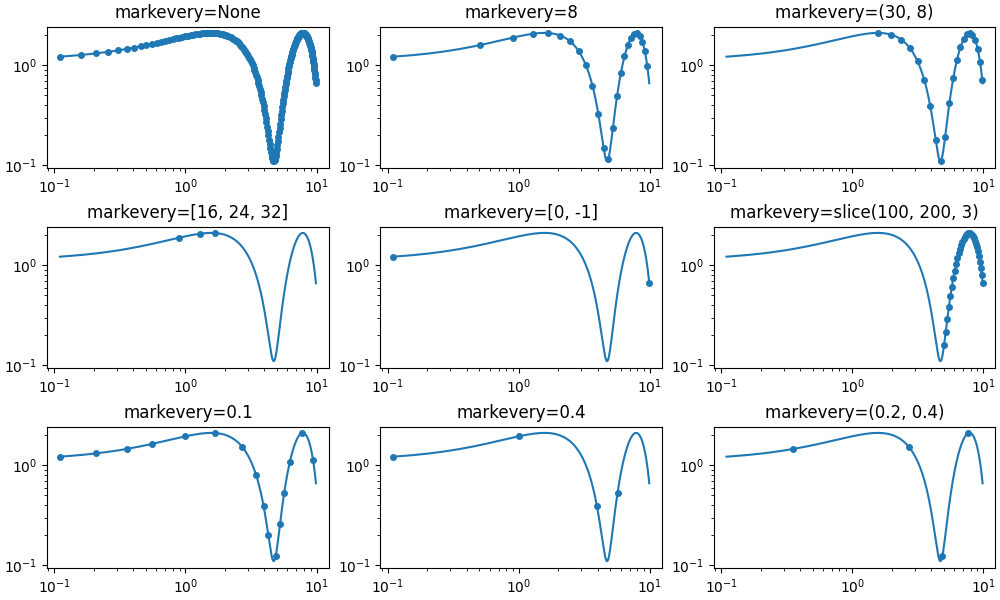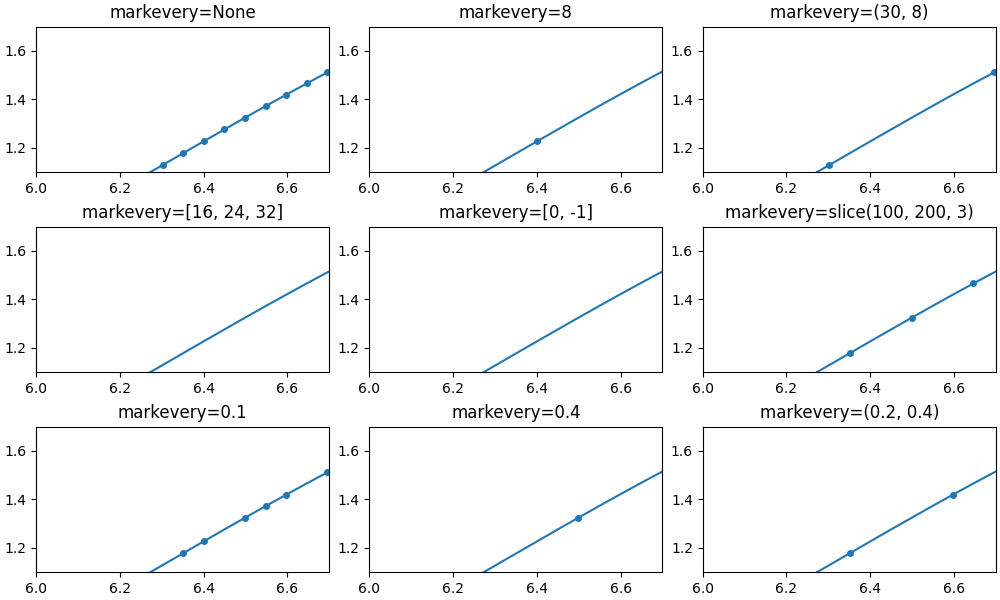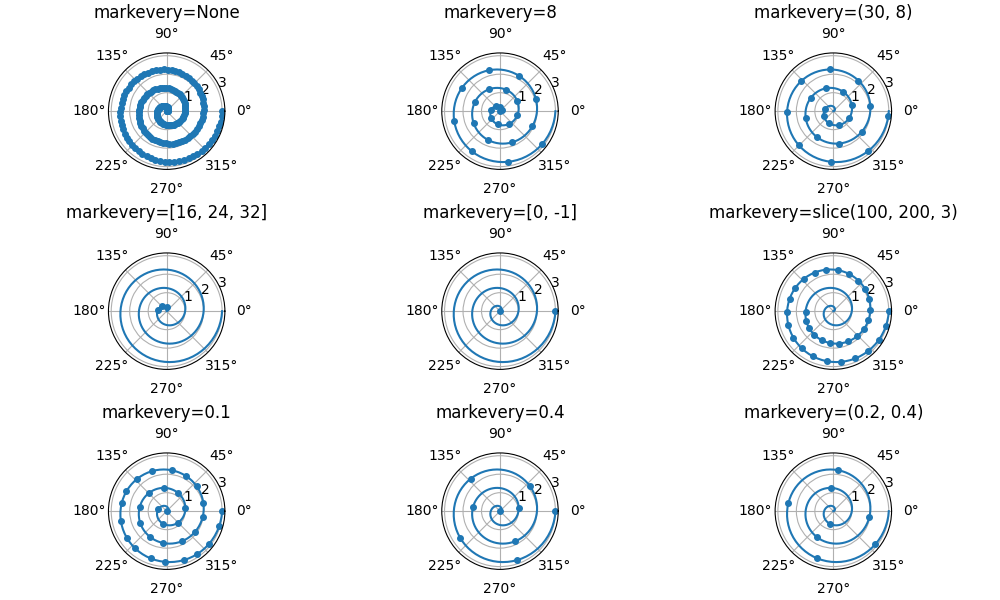# Markevery Demo#

The markevery property of Line2D allows drawing markers at a subset of data points.

The list of possible parameters is specified at Line2D.set_markevery. In short:

• A single integer N draws every N-th marker.

• A tuple of integers (start, N) draws every N-th marker, starting at data index start.

• A list of integers draws the markers at the specified indices.

• A slice draws the markers at the sliced indices.

• A float specifies the distance between markers as a fraction of the Axes diagonal in screen space. This will lead to a visually uniform distribution of the points along the line, irrespective of scales and zooming.

import numpy as np
import matplotlib.pyplot as plt

# define a list of markevery cases to plot
cases = [
None,
8,
(30, 8),
[16, 24, 32],
[0, -1],
slice(100, 200, 3),
0.1,
0.4,
(0.2, 0.4)
]

# data points
delta = 0.11
x = np.linspace(0, 10 - 2 * delta, 200) + delta
y = np.sin(x) + 1.0 + delta


## markevery with linear scales#

fig, axs = plt.subplots(3, 3, figsize=(10, 6), constrained_layout=True)
for ax, markevery in zip(axs.flat, cases):
ax.set_title(f'markevery={markevery}')
ax.plot(x, y, 'o', ls='-', ms=4, markevery=markevery)## markevery with log scales#

Note that the log scale causes a visual asymmetry in the marker distance for when subsampling the data using an integer. In contrast, subsampling on fraction of figure size creates even distributions, because it's based on fractions of the Axes diagonal, not on data coordinates or data indices.

fig, axs = plt.subplots(3, 3, figsize=(10, 6), constrained_layout=True)
for ax, markevery in zip(axs.flat, cases):
ax.set_title(f'markevery={markevery}')
ax.set_xscale('log')
ax.set_yscale('log')
ax.plot(x, y, 'o', ls='-', ms=4, markevery=markevery)## markevery on zoomed plots#

Integer-based markevery specifications select points from the underlying data and are independent on the view. In contrast, float-based specifications are related to the Axes diagonal. While zooming does not change the Axes diagonal, it changes the displayed data range, and more points will be displayed when zooming.

fig, axs = plt.subplots(3, 3, figsize=(10, 6), constrained_layout=True)
for ax, markevery in zip(axs.flat, cases):
ax.set_title(f'markevery={markevery}')
ax.plot(x, y, 'o', ls='-', ms=4, markevery=markevery)
ax.set_xlim((6, 6.7))
ax.set_ylim((1.1, 1.7))## markevery on polar plots#

r = np.linspace(0, 3.0, 200)
theta = 2 * np.pi * r

fig, axs = plt.subplots(3, 3, figsize=(10, 6), constrained_layout=True,
subplot_kw={'projection': 'polar'})
for ax, markevery in zip(axs.flat, cases):
ax.set_title(f'markevery={markevery}')
ax.plot(theta, r, 'o', ls='-', ms=4, markevery=markevery)

plt.show()Total running time of the script: ( 0 minutes 10.114 seconds)

Keywords: matplotlib code example, codex, python plot, pyplot Gallery generated by Sphinx-Gallery